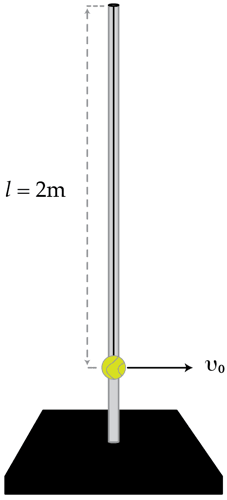# Tetherball

Consider a ball hung from rope of length $l=2~\text{m}$ which is attached to the top of a vertical pole. Initially, the rope is vertical and the ball is given a horizontal initial speed $v_{0}$. What is minimum initial speed $v_{0}$ in meters per second for which the ball hits the top of the pole. Ignore friction, the mass of the rope and treat the ball as a point mass.Details and assumptions

$g=9.8~\text{m}/\text{s}^2$

×